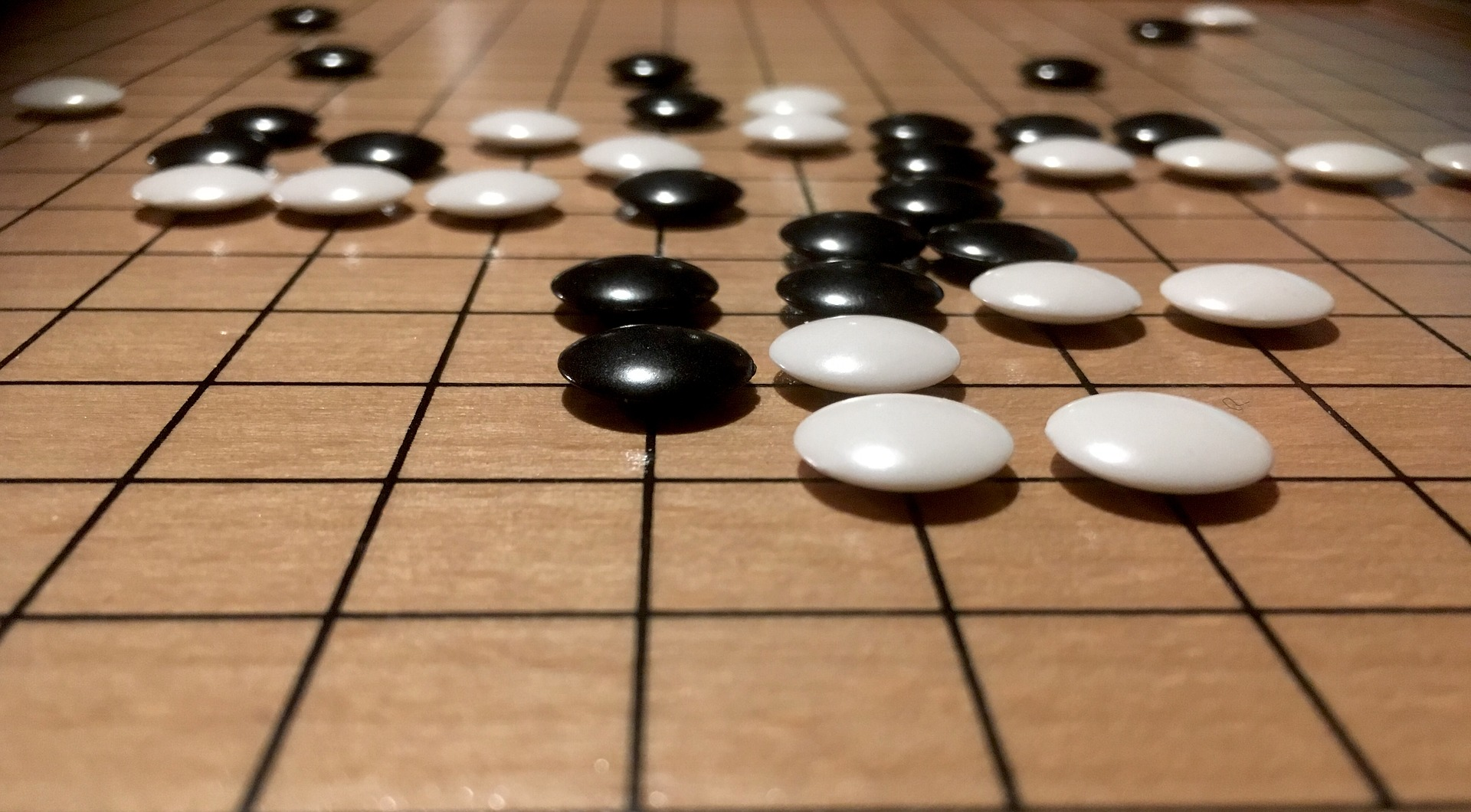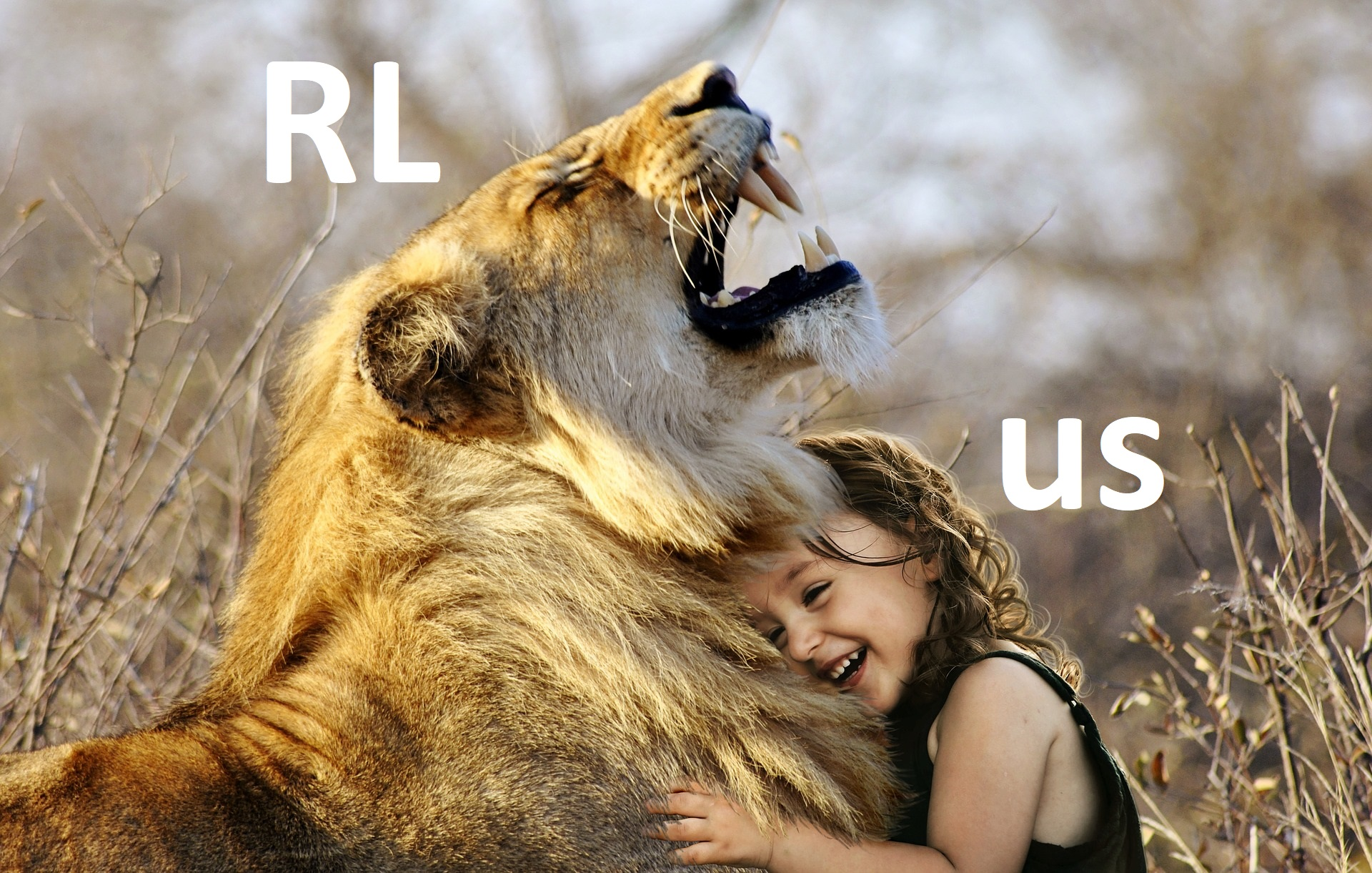# Reinforcement Learning - Goal Oriented Intelligence

Deep Learning Course 4 of 5 - Level: Advanced

## Reinforcement Learning Series Intro - Syllabus Overview

### video

expand_more chevron_left

### text

expand_more chevron_left

### Reinforcement Learning series introduction

What's up, guys? Welcome to this series on reinforcement learning! We'll first start out by introducing the absolute basics to build a solid ground for us to run.We'll then progress onto more advanced and sophisticated topics that integrate neural networks into reinforcement learning. We'll also be getting our hands dirty by implementing some super cool reinforcement learning projects in code! Without further ado, let's get to it!

### What is reinforcement learning?

Reinforcement learning (RL) is an area of machine learning that focuses on how you, or how some thing, might act in an environment in order to maximize some given reward. Reinforcement learning algorithms study the behavior of subjects in such environments and learn to optimize that behavior.

A commonly referred to domain that can illustrate the the power of reinforcement learning is in game playing. I know you've had to come across all the posts and the news and the papers about games that are being played by AI, right?

Take AlphaGo, for example – DeepMind's artificially intelligent Go player that beat the world champion of Go. Even more recently, what's gotten a ton of hype is OpenAI's team of five neural networks, called OpenAI Five, which defeated a team of top professionals in the very complex video game, Dota 2. These human-defeating AIs are being run via reinforcement learning algorithms.So, back to the description of reinforcement learning we mentioned earlier. We said that reinforcement learning focuses on how a given subject might take actions in an environment in order to maximize some reward. Using a game as an example of an environment, reinforcement learning is concerned with how the player of the game can take actions, like making a move in a certain direction, in order to maximize its reward, which in a game setting, might be points.

### The plan

So, what's going to be the approach in this series? Well, if you're anything like me and have tried to jump head first right into reinforcement learning, you may have gotten overwhelmed by all the confusing lingo, unknown acronyms, and abundance of mathematical notation.

I mean, we've got things like Markov Decision Processes, Q-Learning, DQNs, Bellman Equations, Policy Gradients, and not to mention, a bunch of math everywhere that looks like this: Markov Decision Processes, Q-Learning, DQNs, Bellman Equations, Policy Gradients, and not to mention, a bunch of math everywhere that looks like this:

\begin{eqnarray*} v_{\ast }\left( s\right) &=&\max_{a\in \mathbf{A}\left( s\right) }q_{\pi_{\ast }}\left( s,a\right) \\ &=&\max_{a}E_{\pi _{\ast }}\left[ G_{t}\mid S_{t}=s,A_{t}=a\rule[-0.05in]{0in}{0.2in}\right] \\ &=&\max_{a}E_{\pi _{\ast }}\left[ R_{t+1}+\gamma G_{t+1}\mid S_{t}=s,A_{t}=a\rule[-0.05in]{0in}{0.2in}\right] \\ &=&\max_{a}E_{\pi _{\ast }}\left[ R_{t+1}+\gamma v_{\ast }\left(S_{t+1}\right) \mid S_{t}=s,A_{t}=a\rule[-0.05in]{0in}{0.2in}\right] \\ &=&\max_{a}\sum_{s^{\prime },r}p\left( s^{\prime },r\mid s,a\right) \left[r+\gamma v_{\ast }\left( s^{\prime }\right) \rule[-0.05in]{0in}{0.2in}\right] \end{eqnarray*}

Well, fear not! Lucky for us, we're going to tackle this beast of reinforcement learning together step-by-step in a super smooth and intuitive way. As we progress, you'll start to feel more comfortable and confident in your abilities to work with reinforcement learning. Adding this skill to your machine learning toolbox will prove to be immensely useful and valuable!Through this series, we'll get exposure to and gain an understanding of the intuition, the math, and the coding involved with reinforcement learning. Some parts of the series will be purely intuitive, others are going to be more mathematically involved, and then others are going to be more programmatically involved. We'll first focus more on the intuition and some of the math, and then we'll dive into coding reinforcement learning programs.

#### Reinforcement learning series syllabus

Here is the syllabus for parts (1) and (2) of the series. We intend to add more parts and sections as the series progresses. When this happens, we'll update this page, so be sure to take a peek at this for updates every so often.

• Part 1: Introduction to Reinforcement Learning
• Section 1: Markov Decision Processes (MDPs)
• Introduction to MDPs
• Policies and value functions
• Learning optimal policies and value functions
• Section 2: Q-learning
• Introduction to Q-learning with value iteration
• Implementing an epsilon greedy strategy
• Section 3: Code project - Implement Q-learning with pure Python to play a game
• Environment set up and intro to OpenAI Gym
• Write Q-learning algorithm and train agent to play game
• Watch trained agent play game
• Part 2: Deep Reinforcement Learning
• Section 1: Deep Q-networks (DQNs)
• Introduction to DQNs
• Experience replay
• Section 2: Code project - Implement deep Q-network with PyTorch to play a game
• Environment set up
• Create and train DQN to play game
• Watch trained DQN play game
• More details to come
• Part 3 and after: To be announced

If at any point you hit a speed-bump along any of the more technical parts, don't be discouraged! Take a breath, focus, ask questions, and I promise you'll be alright. This stuff takes effort, and we've got you! ❤️

### Resources for getting started

This reinforcement learning series will have plenty of resources that can ensure your success.Here is a list of resources you'll have right at your fingertips:

So, if you're down to follow along with me, drop a comment, and let me know! As you continue through the series, keep me posted with your progress, how your understanding is developing, and what questions you have.

I look forward to hearing from you, and I'll see ya in the next one!

### quiz

expand_more chevron_leftDEEPLIZARD Message notifications

### resources

expand_more chevron_left

expand_more chevron_leftDEEPLIZARD Message notifications